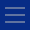## 9月20日讲座：一种更实际的主观决策论

Abstract: In his classic, Savage develops a formal system of rational decision making. It is based on (i) a set of possible states of the world (ii) a set of consequences, (iii) a set of acts, which are functions from states to consequences, and (iv) a preference relation over the acts, which represents the preferences of an idealized rational agent. The goal and the culmination of the enterprise is a representation theorem: Any preference relation that satisfies certain postulates (arguably acceptable on various grounds) determines a (finitely additive) probability distribution over the states and a utility assignment to the consequences, such that the preferences are determined by the expected utilities of the acts. Additional problematic assumptions are however required in Savage's proofs. First, there is a Boolean algebra of events (sets of states) which determines the richness of the set of acts. The probabilities are assigned to members of this algebra. Savage's proof requires that this be a σ-algebra (i.e., closed under infinite countable unions and intersections), which makes for an extremely rich preference relation. On Savage's view we should not require the probability to be σ-additive, hence he finds the insistence on a σ-algebra, peculiar and is somewhat unhappy with it but sees no way of avoiding it. Second, the assignment of utilities requires the constant act assumption: for every consequence there is a constant act, which has that consequence in every state. This is known to be highly counterintuitive. The present work includes two mathematical results. The first, and the more difficult one, shows that the σ-algebra assumption can be dropped. The second states that, as long as utilities are assigned to finite gambles only, the constant act assumption can be replaced by the plausible, much weaker assumption that there are at least two non-equivalent constant acts. The paper discusses the notion of "idealized agent" that underlies Savage's approach, and argues that the simplified system, which is adequate for all the actual purposes for which the system is designed, involves a more realistic notion of an idealized agent.20160920151206_Abstract.pdf
TOP